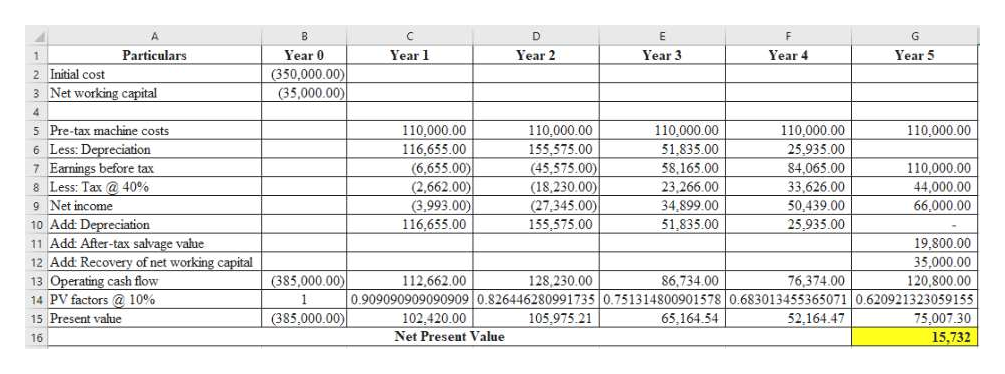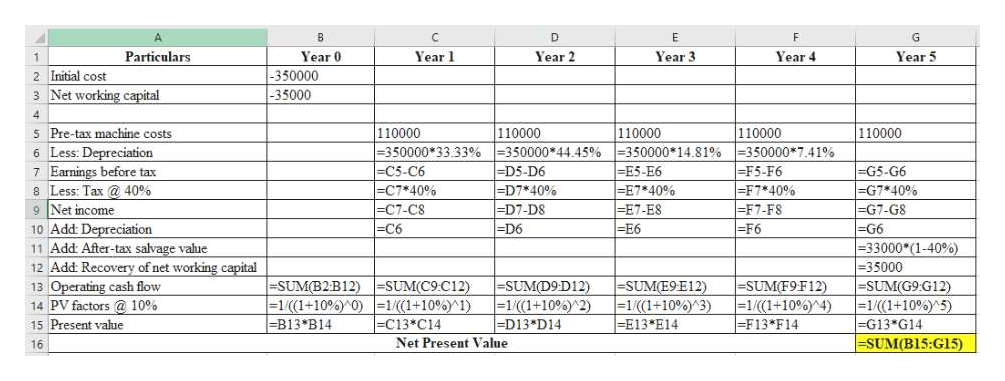Question
211 views

New-Project Analysis

Madison Manufacturing is considering a new machine that costs \$350,000 and would reduce pre-tax manufacturing costs by \$110,000 annually. Madison would use the 3-year MACRS method to depreciate the machine, and management thinks the machine would have a value of \$33,000 at the end of its 5-year operating life. The applicable depreciation rates are 33.33%, 44.45%, 14.81%, and 7.41%. Working capital would increase by \$35,000 initially, but it would be recovered at the end of the project's 5-year life. Madison's marginal tax rate is 40%, and a 10% cost of capital is appropriate for the project.

1. Assume management is unsure about the \$110,000 cost savings - this figure could deviate by as much as plus or minus 20%. Calculate the NPV if cost savings value deviate by plus 20%. Round your answer to the nearest dollar.

Calculate the NPV if cost savings value deviate by minus 20%. Round your answer to the nearest dollar.
\$

2. Suppose the CFO wants you to do a scenario analysis with different values for the cost savings, the machine's salvage value, and the working capital (WC) requirement. She asks you to use the following probabilities and values in the scenario analysis:

 Scenario Probability CostSavings SalvageValue WC Worst case 0.35 \$  88,000 \$28,000 \$40,000 Base case 0.35 110,000 33,000 35,000 Best case 0.30 132,000 38,000 30,000

Calculate the project's expected NPV. Round your answer to the nearest dollar.

Calculate the project's standard deviation. Round your answer to the nearest dollar.

Calculate the project's coefficient of variation. Round your answer to two decimal places.

check_circle

Step 1

As per the authoring guidelines, we should answer first question only if multiple questions posted under single question. Hence, I have answered the first question. Please repost the each question separately.

Step 2

Calculate the net present value if the pre-tax cost is \$110,000 as follows:help_outlineImage TranscriptioncloseP G Particulars Year 1 Year 2 Year 3 Year 5 Year 0 Year 4 1 (350,000.00) (35,000.00) 2 Initial cost 3 Net working capital 5 Pre-tax machine costs 110,000.00 25,935.00 110,000.00 110,000.00 110,000.00 110,000.00 6 Less: Depreciation 7 Earnings before tax 8 Less: Tax@ 40% 116,655.00 155,575.00 51,835.00 (6,655.00) (2,662.00) (3,993.00) 116,655.00 (45,575.00) (18,230.00) 110,000.00 58,165.00 23,266.00 84,065.00 33,626.00 44,000.00 50,439.00 g Net income (27,345.00) 34,899.00 66,000.00 10 Add: Depreciation 11 Add: After-tax salvage value 12 Add: Recovery of net working capital 155,575.00 51,835.00 25,935.00 19,800.00 35,000.00 (385,000.00 13 Operating cash flow 14 PV factors @ 10% 112,662.00 128,230.00 86,734.00 76,374.00 120,800.00 0.909090909090909 0.826446280991735 0.751314800901578 0.683013455365071 0.620921323059155 102.420.00 75,007.30 15 Present value (385,000.00) 105,975.21 65,164.54 52,164.47 Net Present Value 15,732 16 fullscreen
Step 3

Workings...help_outlineImage TranscriptioncloseC P G Year 0 Year 3 Particulars Year Year 2 Year 4 Year 5 -350000 2 Initial cost 3Net working capital -35000 4 5 Pre-tax machine costs 6 Less: Depreciation 7 Earnings before tax 8 Less: Tax @ 40% 9 Net income 110000 - 350000*33.33%- 350000 *44.45% |=D5-D6 =D7*40% =D7-D8 =D6 110000 -350000 14.81% -E5-E6 E7*40% E7-ES -E6 110000 -350000 7.41% |=F5-F6 -F7 40% - F7-FS - F6 110000 110000 |-C5-C6 -C7 40% - C 7 - CS - C6 |-G5-G6 -G7 *40% -G7-G8 -G6 -33000 (1-40%) - 35000 -SUM(G9.G12) - 1 / ( ( 1 + 10 % ) 5 ) |- G13 G14 |-SUM(B15: G15) 10 Add: Depreciation 11 Add: After-tax salvage value 12 Add: Recovery of net working capital SUM(B2B12) -SUM(C9C12) - 1 / ( ( 1 + 10 % ) 0 ) 1 / ( 1+ 10 % ) 1) C13*C14 Net Present Value - SUM(D9 D12 ) -SUM(E9E12 ) - 1 / ( (1 + 10 % ) ^ 3 ) -E13 *E14 - SUM( F9F12 ) |=1/((1+10%)^4) -F13* F14 13 Operating cash flow 14 PV factors @ 10% 15 Present value |= 1 /(1 +10 % ) 2) =D13 D14 - B13 B14 16 fullscreen

### Want to see the full answer?

See Solution

#### Want to see this answer and more?

Solutions are written by subject experts who are available 24/7. Questions are typically answered within 1 hour.*

See Solution
*Response times may vary by subject and question.
Tagged in